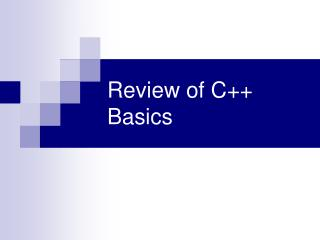Download PresentationReview of C++ BasicsReview of C++ Basics - PowerPoint PPT Presentation

Download PresentationReview of C++ Basics
An Image/Link below is provided (as is) to download presentation

Download Policy: Content on the Website is provided to you AS IS for your information and personal use and may not be sold / licensed / shared on other websites without getting consent from its author. While downloading, if for some reason you are not able to download a presentation, the publisher may have deleted the file from their server.

- - - - - - - - - - - - - - - - - - - - - - - - - - - E N D - - - - - - - - - - - - - - - - - - - - - - - - - - -
Presentation Transcript

1. Review of C++ Basics

2. Problem Solving With Arrays & Functions • Write a program that will read data, sum the numbers, compute the average and find the maximum value • Read data from the console • Read data from a file

3. Program /* Program to process exam grades */ #include <iostream> using namespace std; const int SIZE = 50; /* Function Prototypes */ void readdata(int [ ], int &); int sumarray(int [ ], int); double avgarray(int [ ], int); int findmax(int [ ], int);

4. Main Program int main() { int num; int mark[SIZE]; double avgmark; int numabove,numbelow,numequal,hi_mark; // call function to read the marks and print the array readdata(mark,num); // print the mark array for (count = 0; count < num; count++) cout<<“mark[“<<count<<“]=”<<mark[count] << endl; // find and print the average mark avgmark = avgarray(mark,num); cout <<endl<< "The average is “ << avgmark <<endl; // find and print the highest mark hi_mark = findmax(mark,num); cout << "The highest mark is “ << hi_mark << endl; return 0; }

5. The Function readdata(): /* Function readdata() * Input: numbers - an array to be filled with n numbers * the parameters are uninitialized upon entry * Process: reads n and reads n values from the console into the array * Output:the filled numbers array */ void readdata(int numbers[ ], int &n) { cout << "Enter the number of marks: "; cin >> n; for (int count = 0; count < n; count++) { cout << "Enter a mark: "; cin >> numbers[count]; } return; } Function readdata()

6. Function sumarray() /* Function sumarray() * Input: numbers - an array of n integers * Process: finds the sum of the first n elements in the numbers array. * Output: returns the sum to the calling function. */ int sumarray(int numbers[ ], int n) { int sum=0; for (int count = 0; count < n; count++) sum += numbers[count]; return(sum); }

7. Function avgarray() /* Function avgarray() * Input: numbers - an array of n integers * Process: calls sumarray() to find the sum of the first n elements and then divides by n to find the average. * Output: returns the average to the calling function. */ double avgarray(int numbers[], int n) { return ((double)sumarray(numbers,n)/n); }

8. Finding the Largest Element of an Array /* Function findmax() * Input:numbers - an array of n integers * Process:finds the largest value in the array * Output:returns the maximum value within the array */ int findmax(int numbers[], int n) { int largest_so_far; largest_so_far = numbers; for (int count = 1; count < n; count++) if (largest_so_far < numbers[count]) largest_so_far = numbers[count]; return(largest_so_far); }

9. Reading input data from a file In order to read from a file we need to declare the input file before main() #include <fstream> Ifstream infile; Then in main, open the file so it can be used in the function infile.open(“c://marks.txt”); /* Function readdata() * Input: numbers - an array to be filled with n numbers * the parameters are uninitialized upon entry * Process: reads n values from a file into the array * Output:the filled numbers array */ void readdata(int numbers[ ], int &n) { n=0; while (infile>>numbers[n]) n++; return; }